# Geometry 1 3 Segments Rays and Distance Lines

• Slides: 13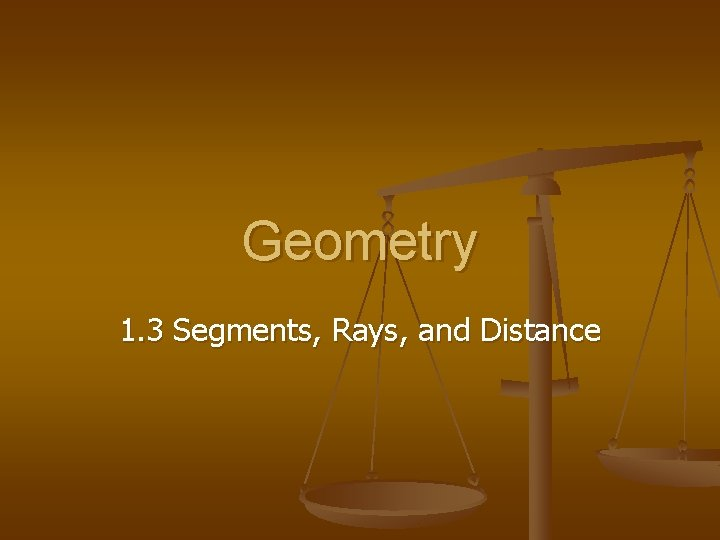Geometry 1. 3 Segments, Rays, and Distance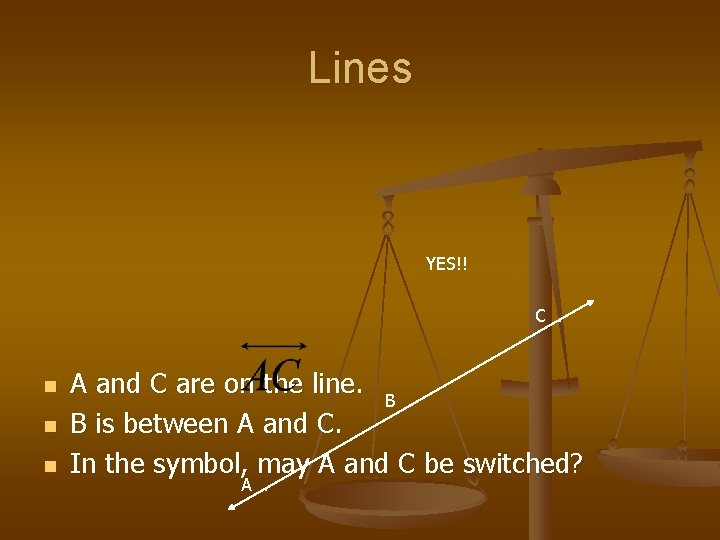Lines YES!! C. n n n A and C are on the line. B. B is between A and C. In the symbol, may A and C be switched? A.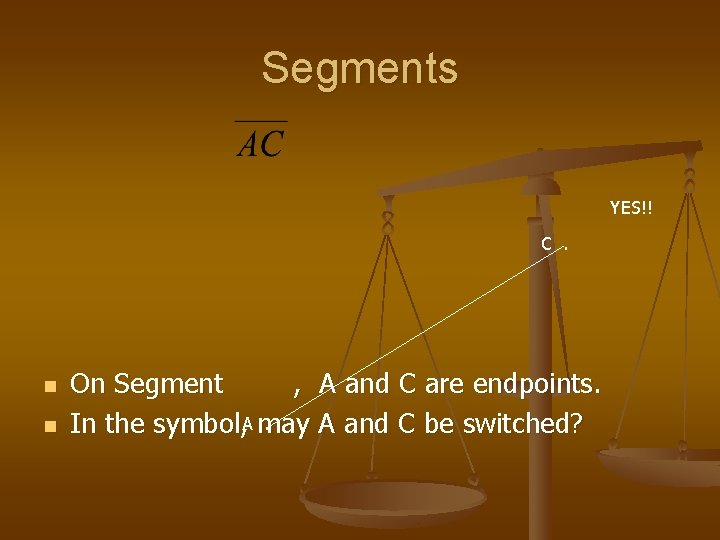Segments YES!! C. n n On Segment , A and C are endpoints. . In the symbol, A may A and C be switched?Rays NO!! n n On ray , A is the endpoint. A. In the symbol, may A and C be switched? C.Opposite Rays . R . S n Ray and ray between R and T. are. opposite rays if S is TLengths C. 4 n n AC, without a symbol, is used to denote the A. length of. AC = 4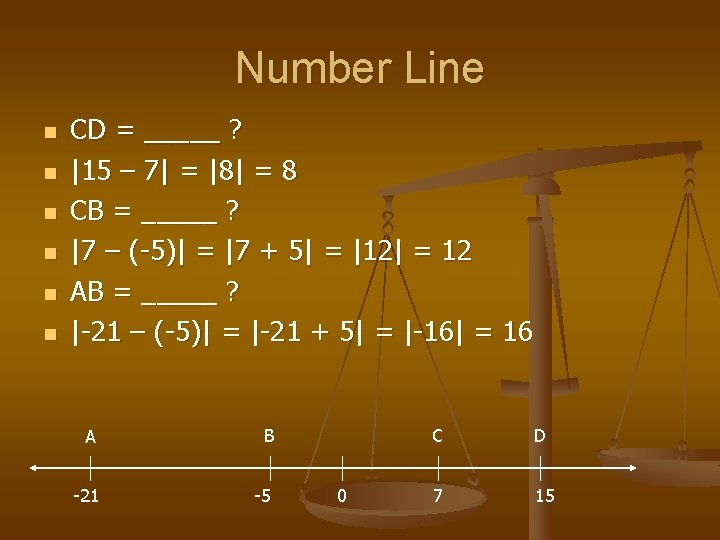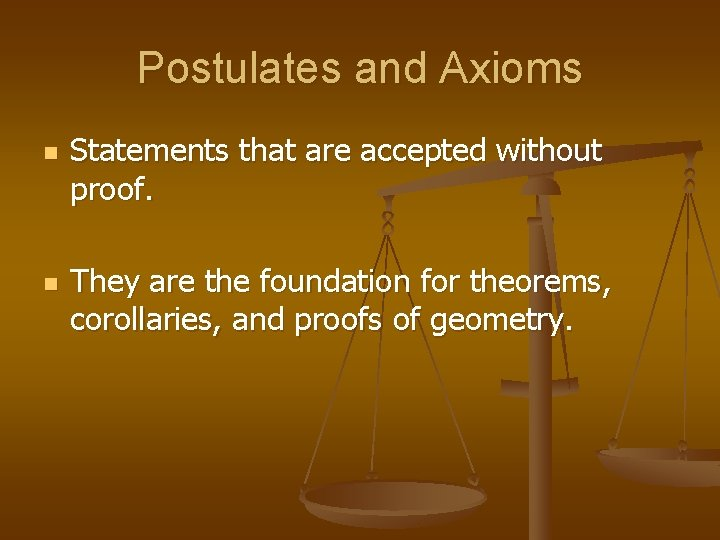Postulates and Axioms n n Statements that are accepted without proof. They are the foundation for theorems, corollaries, and proofs of geometry.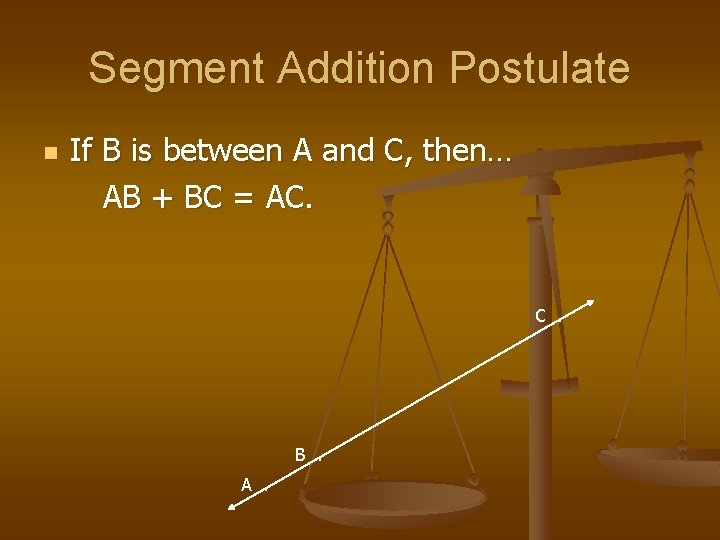Segment Addition Postulate n If B is between A and C, then… AB + BC = AC. B. A.Find x and BC. x + 6 = 24 2 x = 18 x=9 Find BC. BC = x + 6 =9+6 = 15 n AC = 24 . A x . B x+6 . CVocab n n E D . . Congruent objects have the same size and shape. F. DE = FG is equivalent to A Midpoint is the point that divides a segment into two congruent segments. AB M is the midpoint of _____ A bisector of a segment is a line, segment, ray, or plane that intersects a segment at its midpoint. Which segment bisects which segment in the diagram below? XY bisects AB. X. A . M. Y . B G .What have you learned? n n n n Please open your books to P. 15. Let’s answer #6 -#18 even. 6) False 8) True 10) True 12) True 14) False 16) False 18) True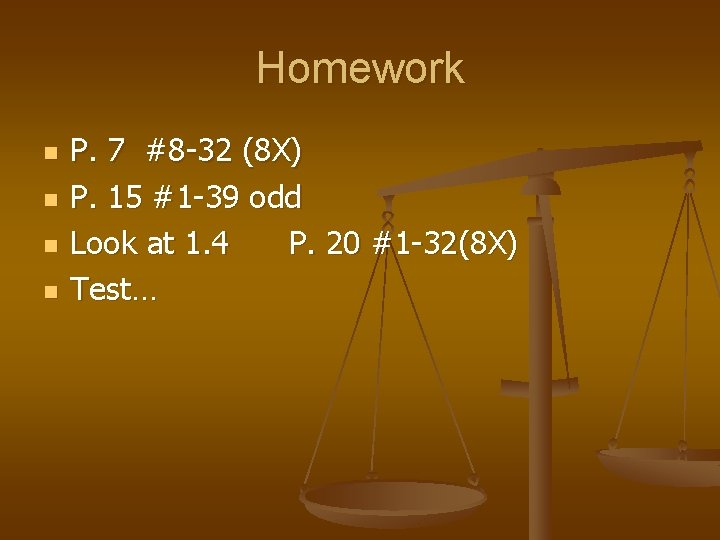Homework n n P. 7 #8 -32 (8 X) P. 15 #1 -39 odd Look at 1. 4 P. 20 #1 -32(8 X) Test…